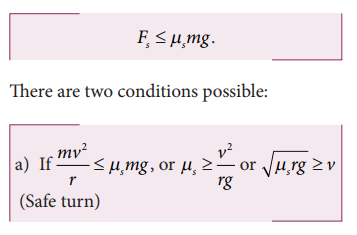Home | | Physics 11th std | Vehicle on a leveled circular road

# Vehicle on a leveled circular road

When a vehicle travels in a curved path, there must be a centripetal force acting on it.

Vehicle on a leveled circular road

When a vehicle travels in a curved path, there must be a centripetal force acting on it. This centripetal force is provided by the frictional force between tyre and surface of the road. Consider a vehicle of mass ‘m’ moving at a speed ‘v’ in the circular track of radius ‘r’. There are three forces acting on the vehicle when it moves as shown in the Figure 3.43

1.        Gravitational force (mg) acting downwards

2.        Normal force (mg) acting upwards

3.        Frictional force (Fs) acting horizontally inwards along the roadSuppose the road is horizontal then the normal force and gravitational force are exactly equal and opposite. The centripetal force is provided by the force of static friction Fs between the tyre and surface of the road which acts towards the center of the circular track,As we have already seen in the previous section, the static friction can increase from zero to a maximum valueThe static friction would be able to provide necessary centripetal force to bend the car on the road. So the coefficient of static friction between the tyre and the surface of the road determines what maximum speed the car can have for safe turn.If the static friction is not able to provide enough centripetal force to turn, the vehicle will start to skid.

## Solved Example Problems for Vehicle on a leveled circular road

### Example 3.24

Consider a circular leveled road of radius 10 m having coefficient of static friction 0.81. Three cars (A, B and C) are travelling with speed 7 ms-1, 8 m s-1 and 10 m s-1 respectively. Which car will skid when it moves in the circular level road? (g =10 m s-2)

### Solution

From the safe turn condition the speed of the vehicle (v) must be less than or equal toThe speed of car A, B and C are 7 m s-1, 8 m s-1 and 10 m s-1 respectively. The cars A and B will have safe turns. But the car C has speed 10 m s-1 while it turns which exceeds the safe turning speed. Hence, the car C will skid.

Study Material, Lecturing Notes, Assignment, Reference, Wiki description explanation, brief detail
11th Physics : UNIT 3 : Laws of Motion : Vehicle on a leveled circular road |